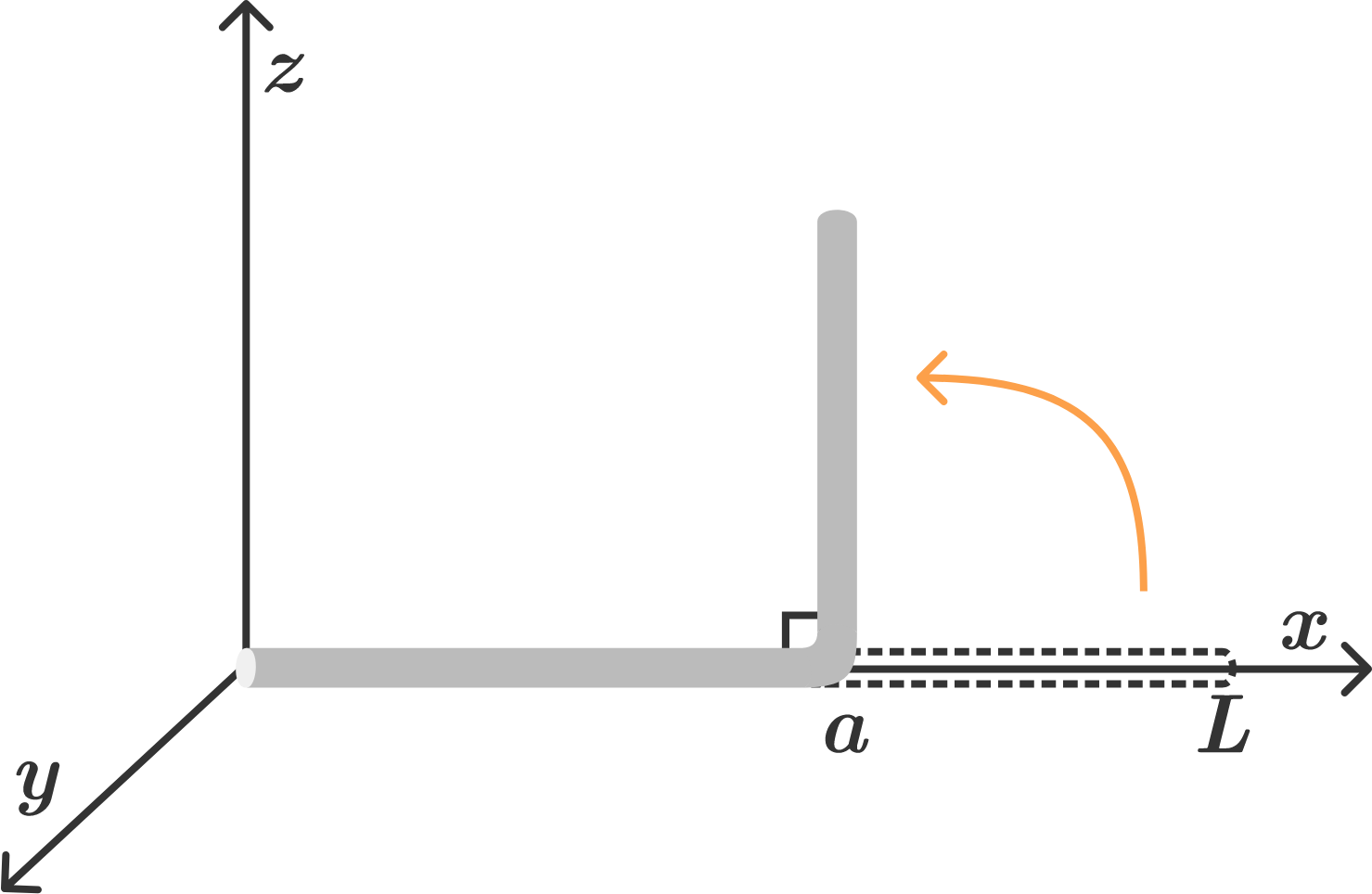# Bent Rod MomentThere is a thin uniform rod of mass $M$ in the $xy$-plane which is initially aligned with the $x$-axis. The body of the rod begins at the origin $x=0$ and ends at $x = L$.

Suppose that the portion of the rod up to $x = a$, where $a < L$ remains aligned with the $x$-axis, and the portion from $x = a$ to $x = L$ is bent upwards at a right angle so as to be perpendicular to the $xy$-plane.

The bent rod's moment of inertia with respect to the $z$-axis can be expressed as ${M a^{2} - \dfrac{\alpha}{\beta} \dfrac{ Ma^{3}}{ L}} .$ If $\alpha$ and $\beta$ are coprime positive integers, determine $\alpha+\beta$.

×

Problem Loading...

Note Loading...

Set Loading...